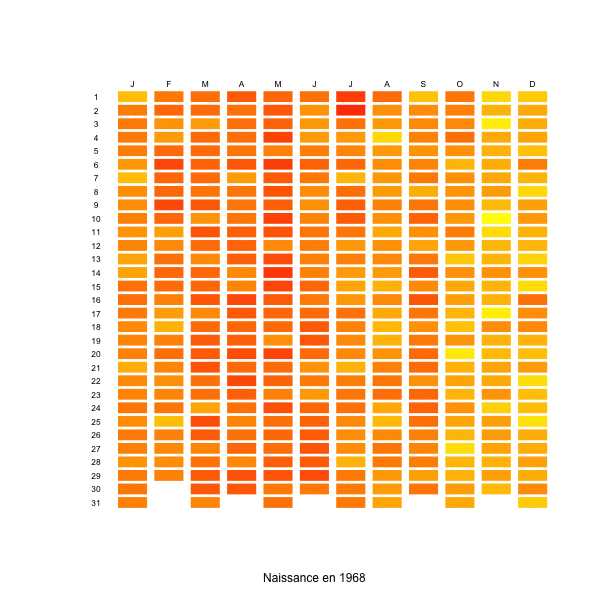# Births and week-ends, in France

This week, I have seen on the internet (sorry, I cannot find proper references) the graph produced here on the right: which birthday is most likely ? The fact that I have no further information is important, since I do not know in which country such a graph was obtained. At least, I know it should not be France…

In France, I have already mentioned that there is a strong week-end effect: nowadays, there is 25% less deliveries during week-ends than during the week. Calot (1981) observed already that there were less deliveries on Sundays. This has been confirmed more recently, e.g. in http://www.lepoint.fr/ or http://www.prepabl.fr/, with a significant difference between week days, and week-ends. Here  is the number of birth per day, over 40 years, with in blue the average trend during the week, and in red, during week-ends,

```naissance=read.table(
"http://freakonometrics.free.fr/naissanceFR2.txt")
attach(naissance)
date=as.Date(date)
plot(date, nbre,cex=.5)
t2=as.POSIXlt(date)
jour=t2\$wday
X=naissance\$date
Y=naissance\$nbre
J=jour
df=data.frame(X,Y,J)
library(splines)
regs=lm(Y~bs(X,df=20),data=df[jour%in%c(0,6),])
Yp=predict(regs,newdata=df)
lines(X,Yp,col="red",lwd=3)
regs=lm(Y~bs(X,df=20),data=df[jour%in%1:5,])
Yp=predict(regs,newdata=df)
lines(X,Yp,col="blue",lwd=3)```If we look at the evolution of the ratio week-ends over weeks days, we have the following graph

```t2=as.POSIXlt(date)
jour=t2\$wday
jour=jour[1:(1982*7)]
nbre2=jour
for(i in 1:1982){
taux=sum(nbre[6:7+7*(i-1)])/
sum(nbre[1:5+7*(i-1)])/2*5
nbre2[1:5+7*(i-1)]=nbre[1:5+7*(i-1)]*taux
nbre2[6:7+7*(i-1)]=nbre[6:7+7*(i-1)]
nbre2[1:7+7*(i-1)]=
mean(nbre[1:7+7*(i-1)])/mean(nbre2[1:7+7*(i-1)])*
nbre2[1:7+7*(i-1)]
}
nbretaux=jour
for(i in 1:1982){
taux=sum(nbre[6:7+7*(i-1)])/
sum(nbre[1:5+7*(i-1)])/2*5
nbretaux[1:7+7*(i-1)]=taux
}
plot(date[1:length(nbre2)],nbretaux)
X= date[1:length(nbre2)]
Y=nbretaux
library(splines)
reg=lm(Y~bs(X,df=20))
Yp=predict(reg)
lines(X,Yp,col="red",lwd=3)```In the beginning of the 70’s, during week-ends, there were 5% less deliveries, but 25% less around 2000. It is then possible to produce the same kind of graphs as the one above, per year of birth. And here, we clearly observe the importance of the week end effect (maybe also because of color choice)

```naissance=read.csv(
"http://freakonometrics.free.fr/naissanceFR.csv",
sep=";")
M=as.matrix(naissance[,3:ncol(naissance)])
BIRTH=as.vector(t(M))
YEAR=rep(1968:2005,each=12*31)
MONTH=rep(rep(1:12,each=31),38)
DAY=rep(1:31,12*38)
X=NA
for(y in 1968:2005){
sbase=base[YEAR==y,]
X=c(X,sbase\$BIRTH/sum(sbase\$BIRTH,
na.rm=TRUE))
}
base=data.frame(YEAR,MONTH,DAY,
BIRTH,BIRTHDAYPROB=X[-1])

m1=min(base\$BIRTHDAYPROB,na.rm=TRUE)
m2=max(base\$BIRTHDAYPROB,na.rm=TRUE)
y=1980
colr=rev(heat.colors(100))
sbase=base[YEAR==y,]
plot(0:1,0:1,col="white",xlim=c(-1,12),
ylim=c(-31,1),axes=FALSE,xlab=
paste("Naissance en",y,sep=" "),ylab="")
for(x in 1:nrow(sbase)){
a=sbase\$MONTH[x];b=sbase\$DAY[x]
polygon(c(a-.9,a-.9,a-.1,a-.1),-c(b-.9,b-.1,
b-.1,b-.9),col=colr[(sbase\$BIRTHDAYPROB[x]-m1)/
(m2-m1)*100],border=NA)
}
text((1:12)-.5,.5,c("J","F","M","A","M","J","J",
"A","S","O","N","D"),cex=.7)
text(-.5,-(1:31)+.5,1:31,cex=.7)```# Lunar cycles and birth rates

Je met en ligne un (court) papier sur l’augmentation du nombre de naissance les jours de pleine lune, Lunar cycles and birth rates: from a full Moon to a first quarter Moon effectObjective The purpose of this study was to examine the influence of the lunar cycle on the frequency of deliveries.

Method This was a time series of 29,385,552 births across 470 lunar cycles that were identified from the French National Institute of Statistics from 1968 to 2005.

Results Using nonparametric smoothing techniques to remove patterns, t-tests validated a significant difference in the number of births on a short window around the full Moon, stress by a significant drop after the first quarter Moon.

Conclusion An analysis of 37 years of data demonstrated a small but statistically significant influence of the lunar cycle on deliveries.